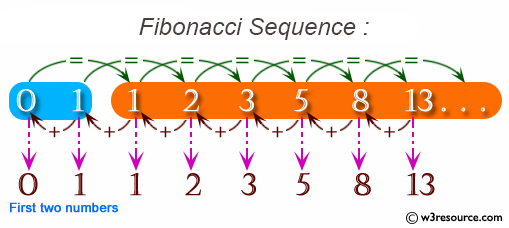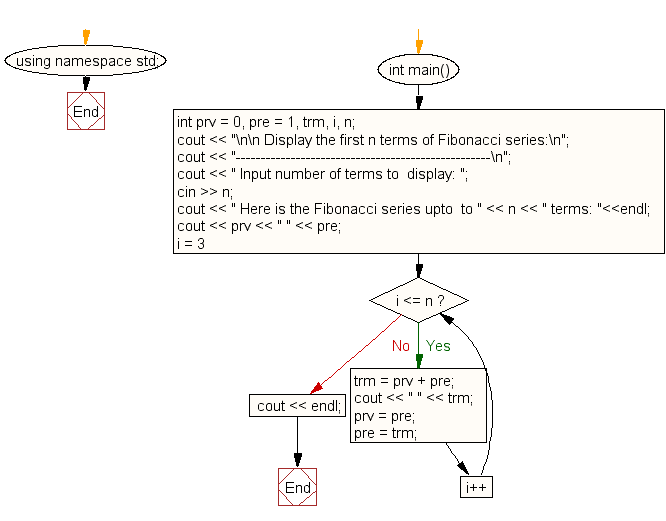﻿ C++ Exercises: Display the first n terms of Fibonacci series - w3resource# C++ Exercises: Display the first n terms of Fibonacci series

## C++ For Loop: Exercise-27 with Solution

Write a program in C++ to display the first n terms of Fibonacci series.

Pictorial Presentation:Sample Solution:-

C++ Code :

``````#include <iostream>
using namespace std;

int main()
{
int prv = 0, pre = 1, trm, i, n;
cout << "\n\n Display the first n terms of Fibonacci series:\n";
cout << "---------------------------------------------------\n";
cout << " Input number of terms to  display: ";
cin >> n;
cout << " Here is the Fibonacci series upto  to " << n << " terms: "<<endl;
cout << prv << " " << pre;
for (i = 3; i <= n; i++)
{
trm = prv + pre;
cout << " " << trm;
prv = pre;
pre = trm;
}
cout << endl;
}
``````

Sample Output:

``` Display the first n terms of Fibonacci series:
---------------------------------------------------
Input number of terms to  display: 10
Here is the Fibonacci series upto  to 10 terms:
0 1 1 2 3 5 8 13 21 34
```

Flowchart:C++ Code Editor:

Contribute your code and comments through Disqus.

What is the difficulty level of this exercise?

﻿

## C++ Programming: Tips of the Day

What is a smart pointer and when should I use one?

This answer is rather old, and so describes what was 'good' at the time, which was smart pointers provided by the Boost library. Since C++11, the standard library has provided sufficient smart pointers types, and so you should favour the use of std::unique_ptr, std::shared_ptr and std::weak_ptr.

There was also std::auto_ptr. It was very much like a scoped pointer, except that it also had the "special" dangerous ability to be copied - which also unexpectedly transfers ownership.

It was deprecated in C++11 and removed in C++17, so you shouldn't use it.

```std::auto_ptr<MyObject> p1 (new MyObject());
std::auto_ptr<MyObject> p2 = p1; // Copy and transfer ownership.
// p1 gets set to empty!
p2->DoSomething(); // Works.
p1->DoSomething(); // Oh oh. Hopefully raises some NULL pointer exception.
```

Ref : https://bit.ly/3mc9GHE